# Chapter 10 Legends

Like the x- or y-axis, a legend is a guide: it shows people how to map visual (aesthetic) properties back to data values.

## 10.1 Removing the Legend

### 10.1.1 Problem

You want to remove the legend from a graph.

### 10.1.2 Solution

Use `guides()`, and specify the scale that should have its legend removed (Figure 10.1):

``````# Create the base plot (with legend)
pg_plot <- ggplot(PlantGrowth, aes(x = group, y = weight, fill = group)) +
geom_boxplot()

pg_plot

# Remove the legend for fill
pg_plot +
guides(fill = FALSE)``````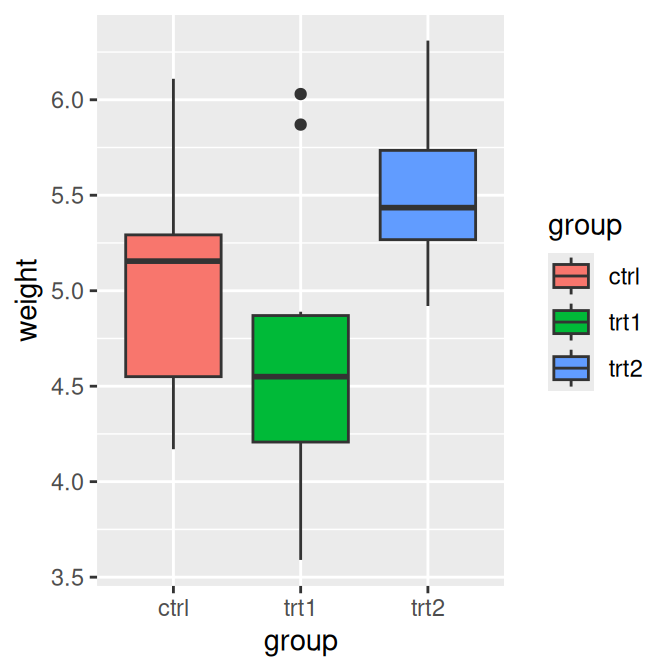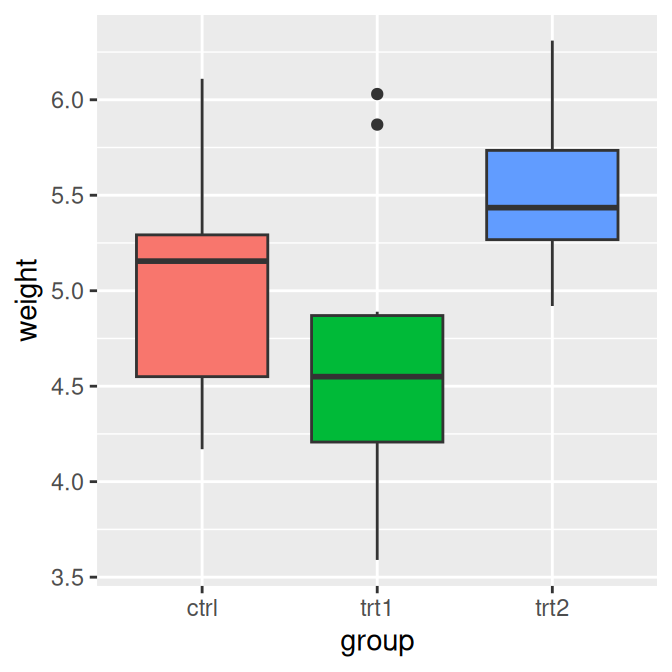Figure 10.1: Default appearance (left); With legend removed (right)

### 10.1.3 Discussion

Another way to remove a legend is to set `guide = FALSE` in the scale. This will result in the exact same output as the preceding code:

``````# Remove the legend for fill
pg_plot +
scale_fill_discrete(guide = FALSE)``````

Yet another way to remove the legend is to use the theming system. If you have more than one aesthetic mapping with a legend (`color` and `shape`, for example), this will remove legends for all of them:

``````pg_plot +
theme(legend.position = "none")``````

Sometimes a legend is redundant, or it is supplied in another graph that will be displayed with the current one. In these cases, it can be useful to remove the legend from a graph.

In the example used here, the colors provide the same information that is on the x-axis, so the legend is unnecessary. Notice that with the legend removed, the area used for graphing the data is larger. If you want to achieve the same proportions in the graphing area, you will need to adjust the overall dimensions of the graph.

When a variable is mapped to `fill`, the default scale used is `scale_fill_discrete()` (equivalent to `scale_fill_hue()`), which maps the factor levels to colors that are equally spaced around the color wheel. There are other scales for `fill`, such as `scale_fill_manual()`. If you use scales for other aesthetics, such as `colour` (for lines and points) or `shape` (for points), you must use the appropriate scale. Commonly used scales include:

• `scale_fill_discrete()`
• `scale_fill_hue()`
• `scale_fill_manual()`
• `scale_fill_grey()`
• `scale_fill_brewer()`
• `scale_colour_discrete()`
• `scale_colour_hue()`
• `scale_colour_manual()`
• `scale_colour_grey()`
• `scale_colour_brewer()`
• `scale_shape_manual()`
• `scale_linetype()`

## 10.2 Changing the Position of a Legend

### 10.2.1 Problem

You want to move the legend from its default place on the right side.

### 10.2.2 Solution

Use `theme(legend.position = ...)`. It can be put on the top, left, right, or bottom by using one of those strings as the position (Figure 10.2, left).

``````pg_plot <- ggplot(PlantGrowth, aes(x = group, y = weight, fill = group)) +
geom_boxplot() +
scale_fill_brewer(palette = "Pastel2")

pg_plot +
theme(legend.position = "top")``````

The legend can also be placed inside the plotting area by specifying a coordinate position, as in `legend.position = c(.8, .3)` (Figure 10.2, right). The coordinate space starts at (0, 0) in the bottom left and goes to (1, 1) in the top right.

``````pg_plot +
theme(legend.position = c(.8, .3))``````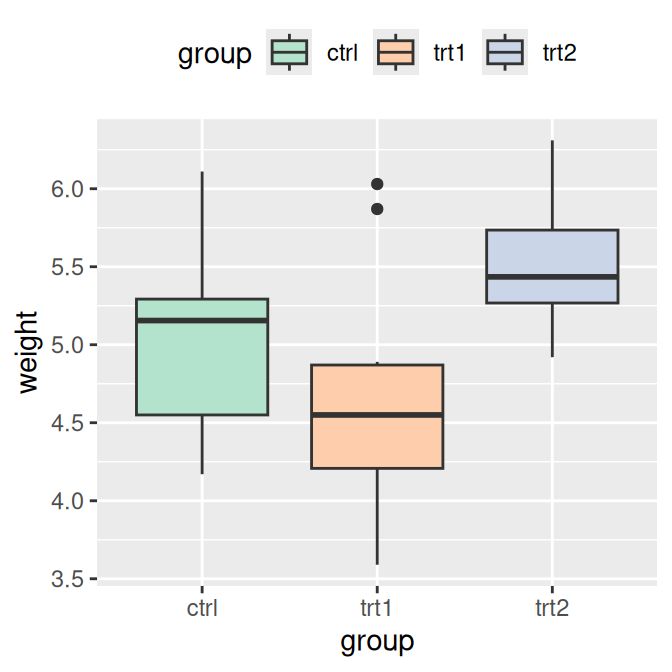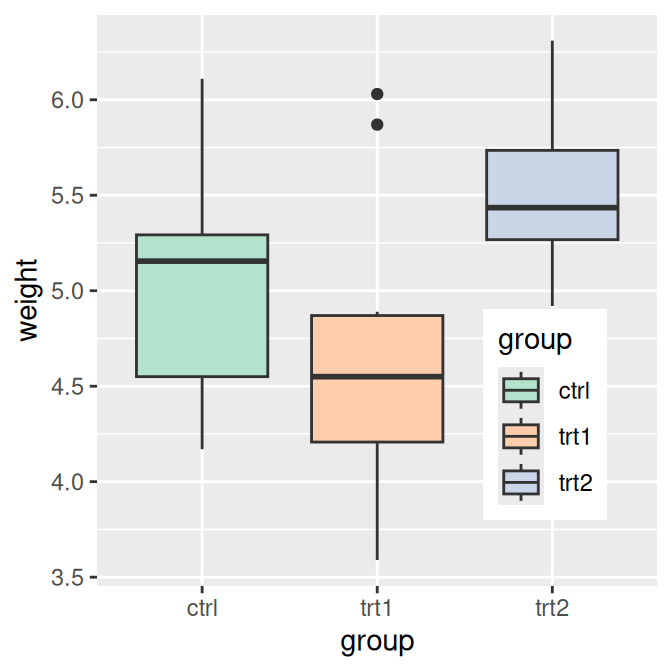Figure 10.2: Legend on top (left); Legend inside of plotting area (right)

### 10.2.3 Discussion

You can also use `legend.justification` to set which part of the legend box is set to the position at `legend.position`. By default, the center of the legend (.5, .5) is placed at the coordinate, but it is often useful to specify a different point.

For example, this will place the bottom-right corner of the legend (1, 0) in the bottom-right corner of the plotting area (1, 0) (Figure 10.3, left):

``````pg_plot +
theme(legend.position = c(1, 0), legend.justification = c(1, 0))``````

And this will place the top-right corner of the legend in the top-right corner of the graphing area (Figure 10.3, right):

``````pg_plot +
theme(legend.position = c(1, 1), legend.justification = c(1, 1))``````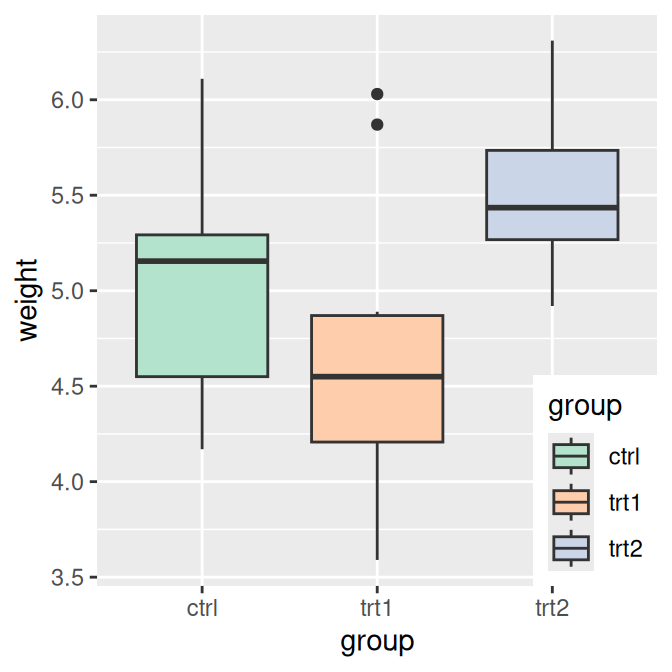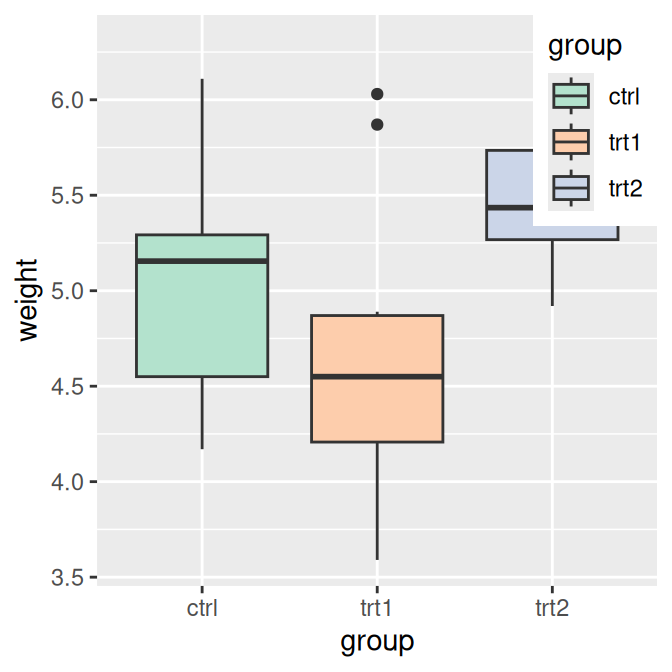Figure 10.3: Legend in bottom-right corner (left); Legend in top-right corner (right)

When placing the legend inside of the graphing area, it may be helpful to add an opaque border to set it apart (Figure 10.4, left):

``````pg_plot +
theme(legend.position = c(.85, .2)) +
theme(legend.background = element_rect(fill = "white", colour = "black"))``````

You can also remove the border around its elements so that it blends in (Figure 10.4, right):

``````pg_plot +
theme(legend.position = c(.85, .2)) +
theme(legend.background = element_blank()) +  # Remove overall border
theme(legend.key = element_blank())           # Remove border around each item``````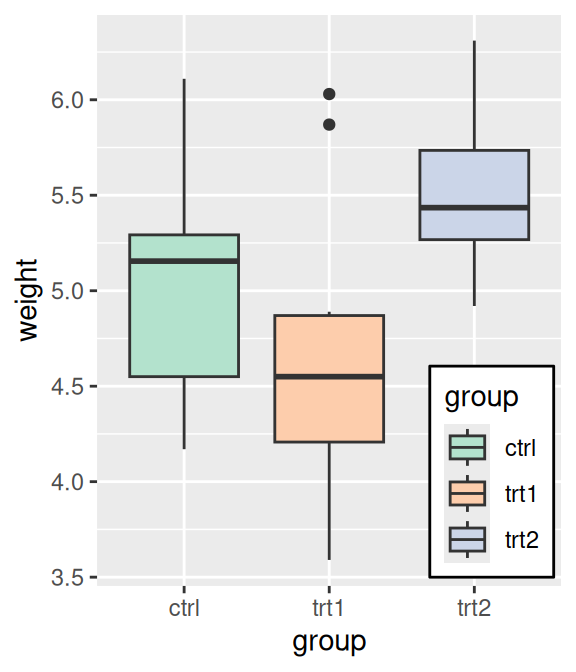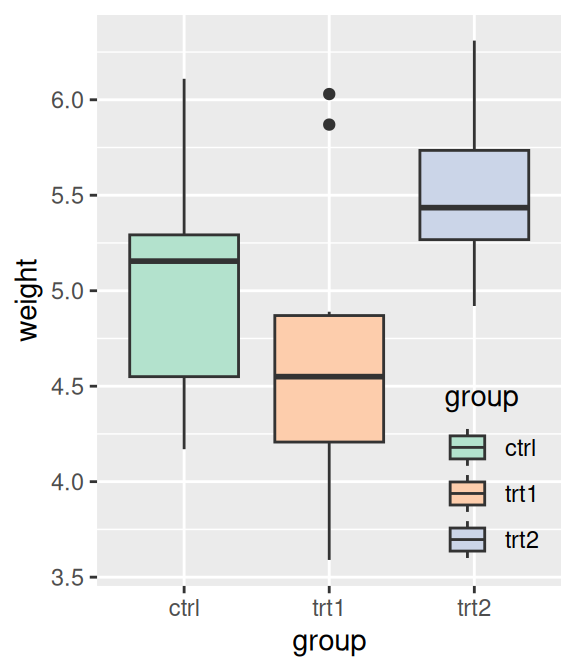Figure 10.4: Legend with opaque background and outline (left); With no background or outlines (right)

## 10.3 Changing the Order of Items in a Legend

### 10.3.1 Problem

You want to change the order of the items in a legend.

### 10.3.2 Solution

Set the limits in the scale to the desired order (Figure 10.5):

``````# Create the base plot
pg_plot <- ggplot(PlantGrowth, aes(x = group, y = weight, fill = group)) +
geom_boxplot()

pg_plot

# Change the order of items
pg_plot +
scale_fill_discrete(limits = c("trt1", "trt2", "ctrl"))``````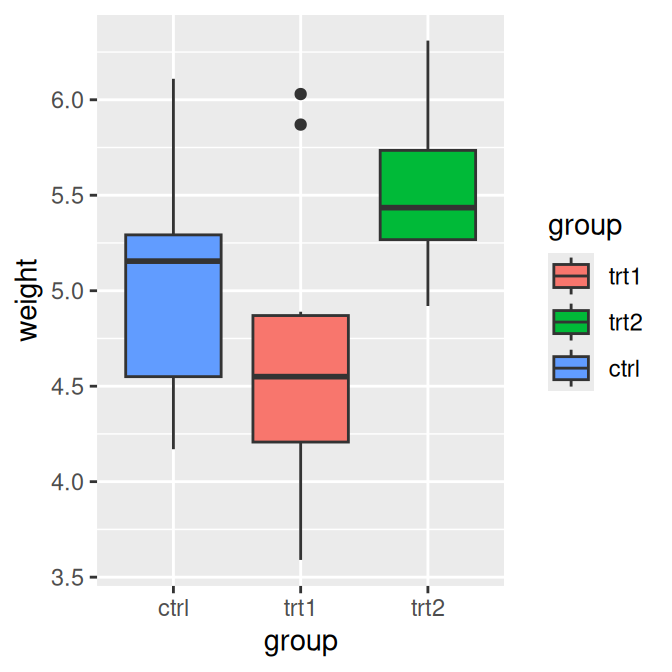Figure 10.5: Default order for legend (left); Modified order (right)

### 10.3.3 Discussion

Note that the order of the items on the x-axis did not change. To do that, you would have to set the limits of `scale_x_discrete()` (Recipe 8.4), or change the data to have a different factor level order (Recipe 15.8).

In the preceding example, group was mapped to the fill aesthetic. By default this uses `scale_fill_discrete()` (which is the sameas `scale_fill_hue()`), which maps the factor levels to colors that are equally spaced around the color wheel. We could have used a different `scale_fill_``xxx``()`, though. For example, we could use a grey palette (Figure 10.6, left):

``````pg_plot +
scale_fill_grey(start = .5, end = 1, limits = c("trt1", "trt2", "ctrl"))``````

Or we could use a palette from RColorBrewer (Figure 10.6, right):

``````pg_plot +
scale_fill_brewer(palette = "Pastel2", limits = c("trt1", "trt2", "ctrl"))``````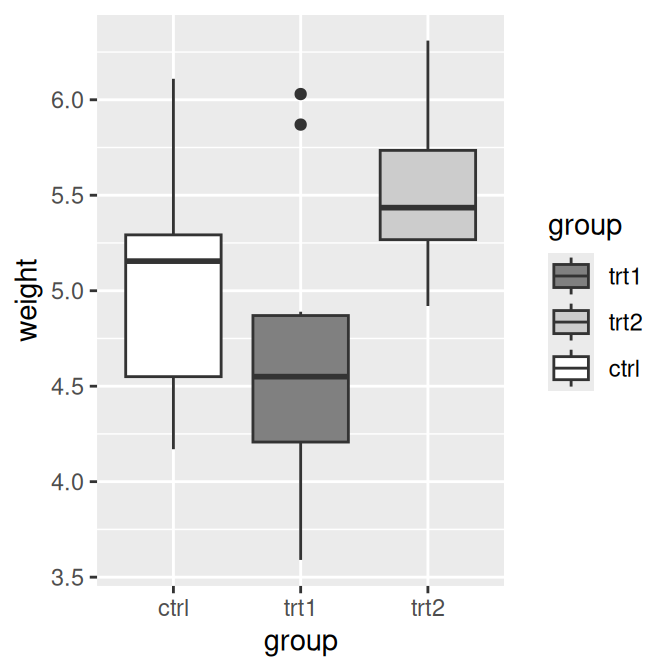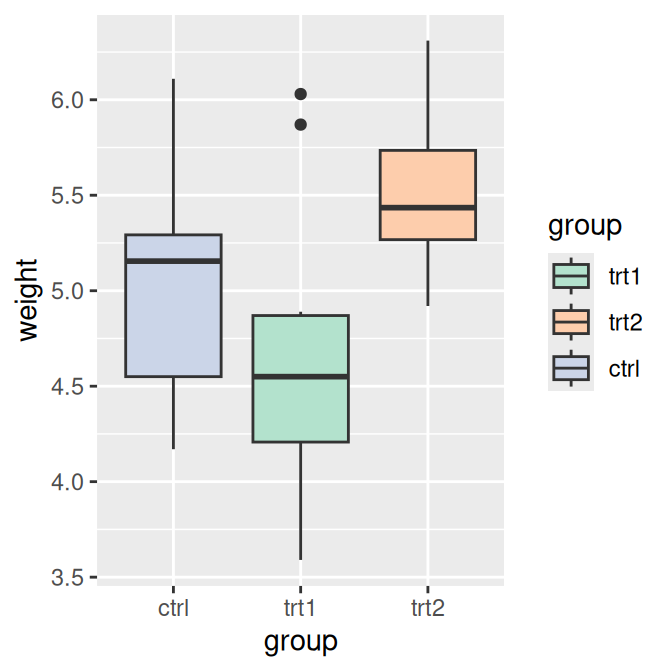Figure 10.6: Modified order with a grey palette (left); With a palette from RColorBrewer (right)

All the previous examples were for fill. If you use scales for other aesthetics, such as colour (for lines and points) or shape (for points), you must use the appropriate scale. Commonly used scales include:

• `scale_fill_discrete()`
• `scale_fill_hue()`
• `scale_fill_manual()`
• `scale_fill_grey()`
• `scale_fill_brewer()`
• `scale_colour_discrete()`
• `scale_colour_hue()`
• `scale_colour_manual()`
• `scale_colour_grey()`
• `scale_colour_brewer()`
• `scale_shape_manual()`
• `scale_linetype()`

By default, using `scale_fill_discrete()` is equivalent to using `scale_fill_hue()`; the same is true for color scales.

### 10.3.4 See Also

To reverse the order of the legend, see Recipe 10.4.

To change the order of factor levels, see Recipe 15.8. To order legend items based on values in another variable, see Recipe 15.9.

## 10.4 Reversing the Order of Items in a Legend

### 10.4.1 Problem

You want to reverse the order of items in a legend.

### 10.4.2 Solution

Add guides (`fill = guide_legend(reverse = TRUE)`) to reverse the order of the legend, as in Figure 10.7 (for other aesthetics, replace `fill` with the name of the aesthetic, such as `colour` or `size`):

``````# Create the base plot
pg_plot <- ggplot(PlantGrowth, aes(x = group, y = weight, fill = group)) +
geom_boxplot()

pg_plot

# Reverse the legend order
pg_plot +
guides(fill = guide_legend(reverse = TRUE))``````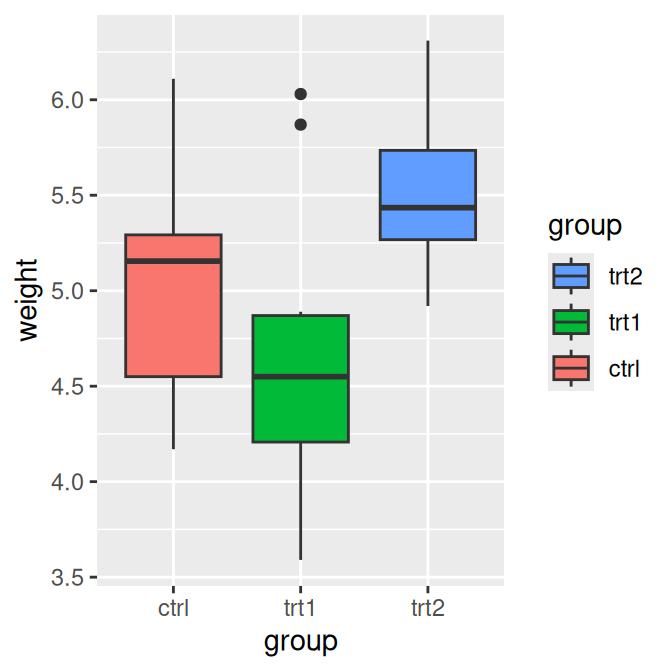Figure 10.7: Default order for legend (left); Reversed order (right)

### 10.4.3 Discussion

It is also possible to control the legend when specifying the scale, as in the following:

``scale_fill_hue(guide = guide_legend(reverse = TRUE))``

## 10.5 Changing a Legend Title

### 10.5.1 Problem

You want to change the text of a legend title.

### 10.5.2 Solution

Use `labs()` and set the value of `fill`, `colour`, `shape`, or whatever aesthetic is appropriate for the legend (Figure 10.8):

``````# Create the base plot
pg_plot <- ggplot(PlantGrowth, aes(x = group, y = weight, fill = group)) +
geom_boxplot()

pg_plot

# Set the legend title to "Condition"
pg_plot + labs(fill = "Condition")``````Figure 10.8: With the legend title set to “Condition”

### 10.5.3 Discussion

It’s also possible to set the title of the legend in the scale specification. Since legends and axes are both guides, this works the same way as setting the title of the x- or y-axis.

This would have the same effect as the previous code:

``pg_plot + scale_fill_discrete(name = "Condition")``

If there are multiple variables mapped to aesthetics with a legend (those other than x and y), you can set the title of each individually. In the example here we’ll use `\n` to add a line break in one of the titles (Figure 10.9):

``````library(gcookbook)  # Load gcookbook for the heightweight data set

# Load gcookbook for the heightweight data set
hw_plot <- ggplot(heightweight, aes(x = ageYear, y = heightIn, colour = sex)) +
geom_point(aes(size = weightLb)) +
scale_size_continuous(range = c(1, 4))

hw_plot

# With new legend titles
hw_plot +
labs(colour = "Male/Female", size = "Weight\n(pounds)")``````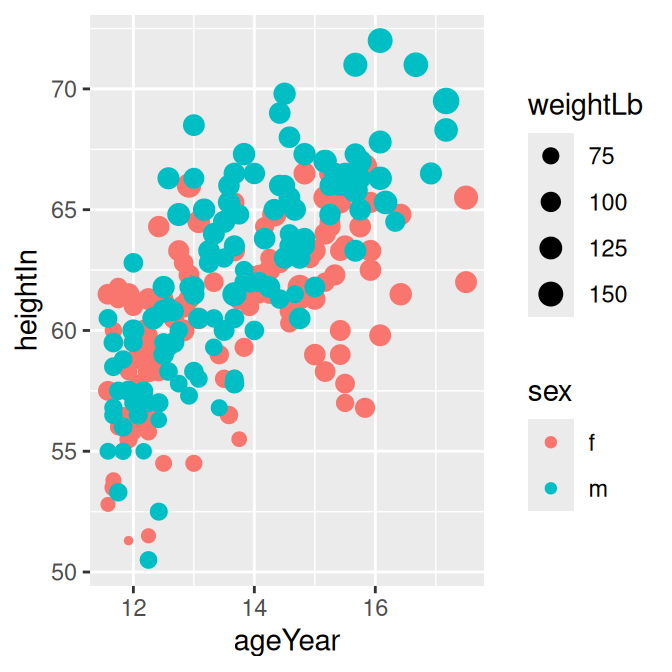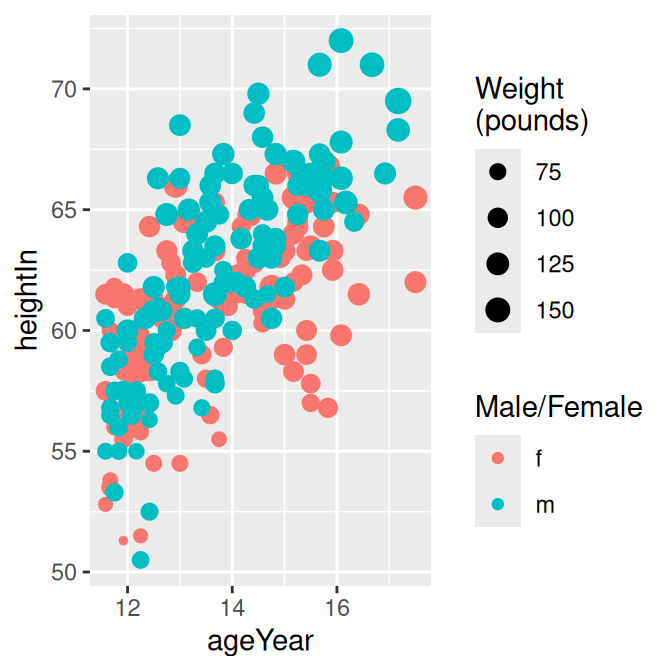Figure 10.9: Two legends with original titles (left); With new titles (right)

If you have one variable mapped to two separate aesthetics, the default is to have a single legend that combines both. For example, if we map `sex` to both `shape` and `weight`, there will be just one legend (Figure 10.10, left):

``````hw_plot2 <- ggplot(heightweight, aes(x = ageYear, y = heightIn, shape = sex, colour = sex)) +
geom_point()

hw_plot2``````

To change the title (Figure 10.10, right), you need to set the name for both of them. If you change the name for just one, it will result in two separate legends (Figure 10.10, middle):

``````# Change just shape
hw_plot2 +
labs(shape = "Male/Female")

# Change both shape and colour
hw_plot2 +
labs(shape = "Male/Female", colour = "Male/Female")``````

It is also possible to control the legend title with the `guides()` function. It’s a little more verbose, but it can be useful when you’re already using it to control other properties:

``````hw_plot +
guides(fill = guide_legend(title = "Condition"))``````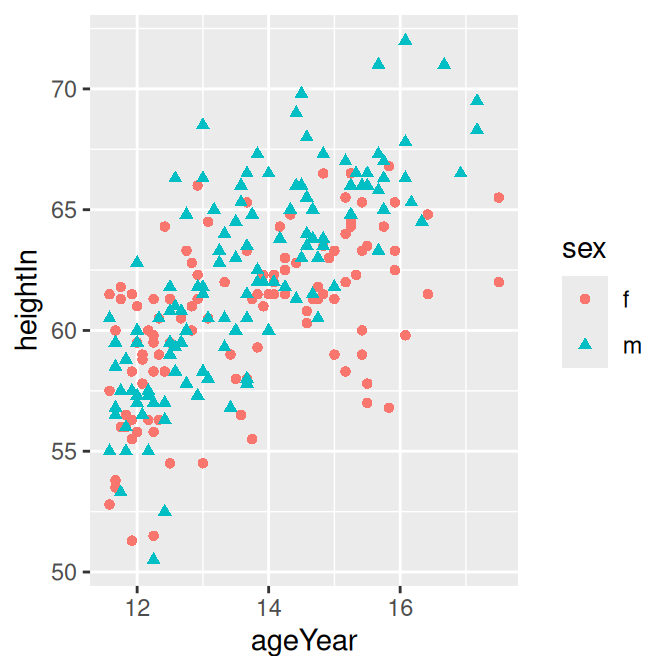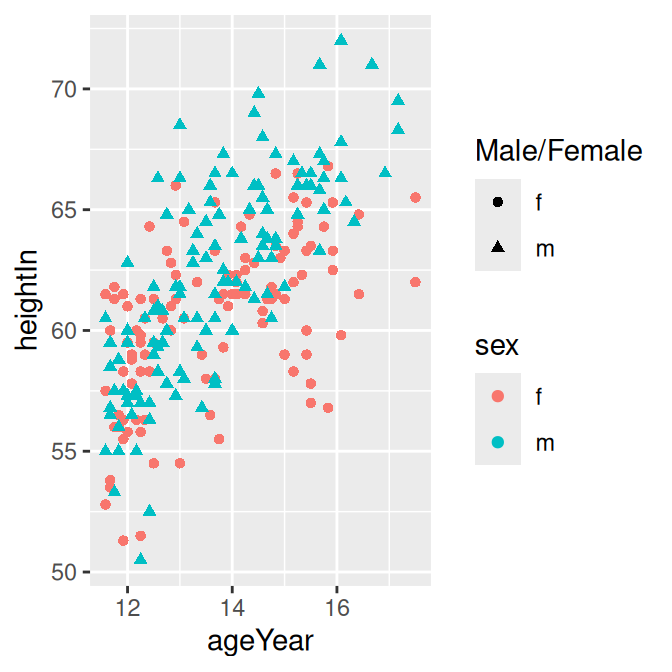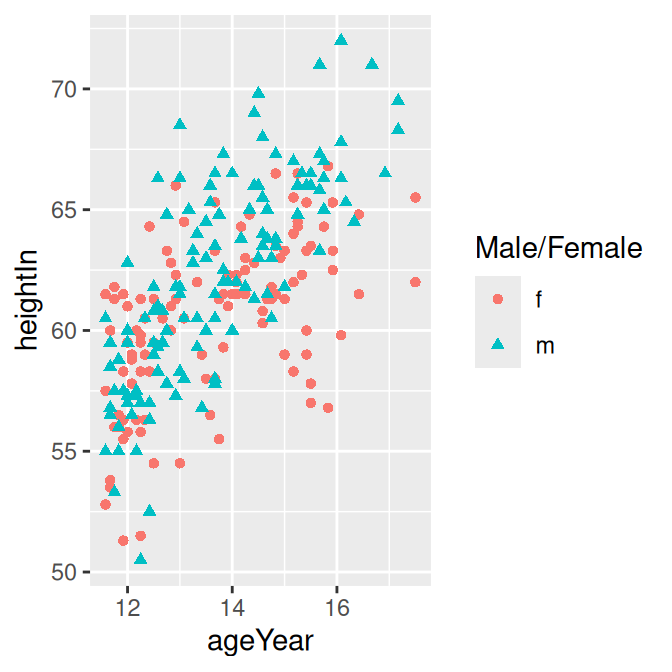Figure 10.10: Default legend with a variable mapped to shape and colour (left); With shape renamed (middle); With both shape and colour renamed (right)

## 10.6 Changing the Appearance of a Legend Title

### 10.6.1 Problem

You want to change the appearance of a legend title’s text.

### 10.6.2 Solution

Use theme(`legend.title = element_text()`) (Figure 10.11):

``````pg_plot <- ggplot(PlantGrowth, aes(x = group, y = weight, fill = group)) +
geom_boxplot()

pg_plot +
theme(legend.title = element_text(
face = "italic",
family = "Times",
colour = "red",
size = 14)
)``````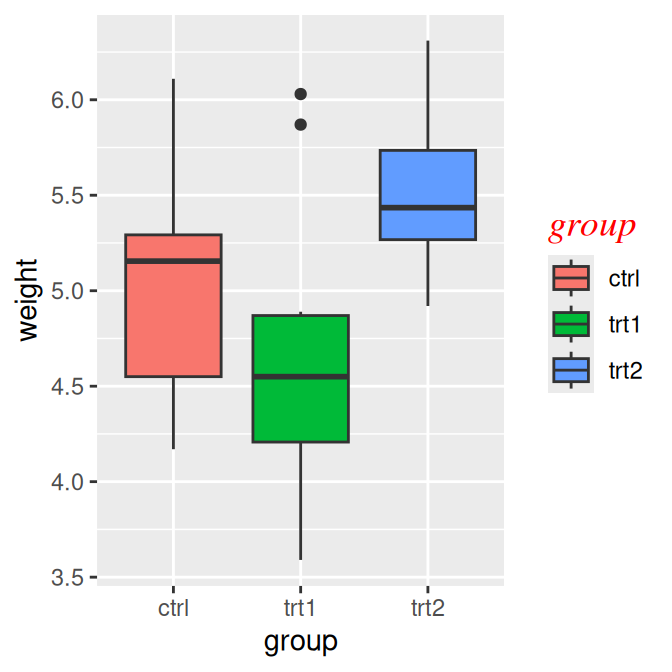Figure 10.11: Customized legend title appearance

### 10.6.3 Discussion

It’s also possible to specify the legend title’s appearance via `guides()`, but this method can be a bit verbose. This has the same effect as the previous code:

``````pg_plot +
guides(fill = guide_legend(title.theme = element_text(
face = "italic",
family = "times",
colour = "red",
size = 14))
)``````

### 10.6.4 See Also

See Recipe 9.2 for more on controlling the appearance of text.

## 10.7 Removing a Legend Title

### 10.7.1 Problem

You want to remove a legend title.

### 10.7.2 Solution

Add `guides(fill = guide_legend(title = NULL))` to remove the title from a legend, as in Figure 10.12 (for other aesthetics, replace `fill` with the name of the aesthetic, such as `colour` or `size`):

``````ggplot(PlantGrowth, aes(x = group, y = weight, fill = group)) +
geom_boxplot() +
guides(fill = guide_legend(title = NULL))``````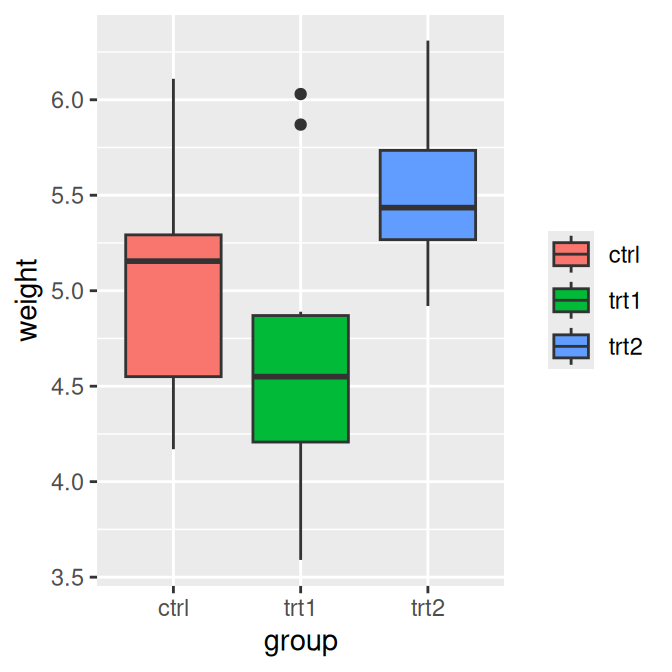Figure 10.12: Box plot with no legend title

### 10.7.3 Discussion

It is also possible to control the legend title when specifying the scale. This has the same effect as the preceding code:

``scale_fill_hue(guide = guide_legend(title = NULL))``

## 10.8 Changing the Labels in a Legend

### 10.8.1 Problem

You want to change the text of labels in a legend.

### 10.8.2 Solution

Set the labels in the scale (Figure 10.13, left):

``````library(gcookbook)  # Load gcookbook for the PlantGrowth data set

# The base plot
pg_plot <- ggplot(PlantGrowth, aes(x = group, y = weight, fill = group)) +
geom_boxplot()

# Change the legend labels
pg_plot +
scale_fill_discrete(labels = c("Control", "Treatment 1", "Treatment 2"))``````

### 10.8.3 Discussion

Note that the labels on the x-axis did not change. To do that, you would have to set the labels of `scale_x_discrete()` (Recipe 8.10), or change the data to have different factor level names (Recipe 15.10).

In the preceding example, group was mapped to the fill aesthetic. By default this uses `scale_fill_discrete()`, which maps the factor levels to colors that are equally spaced around the color wheel (the same as `scale_fill_hue()`). There are other fill scales we could use, and setting the labels works the same way. For example, to produce the graph on the right in Figure 10.13:

``````pg_plot +
scale_fill_grey(start = .5, end = 1, labels = c("Control", "Treatment 1", "Treatment 2"))``````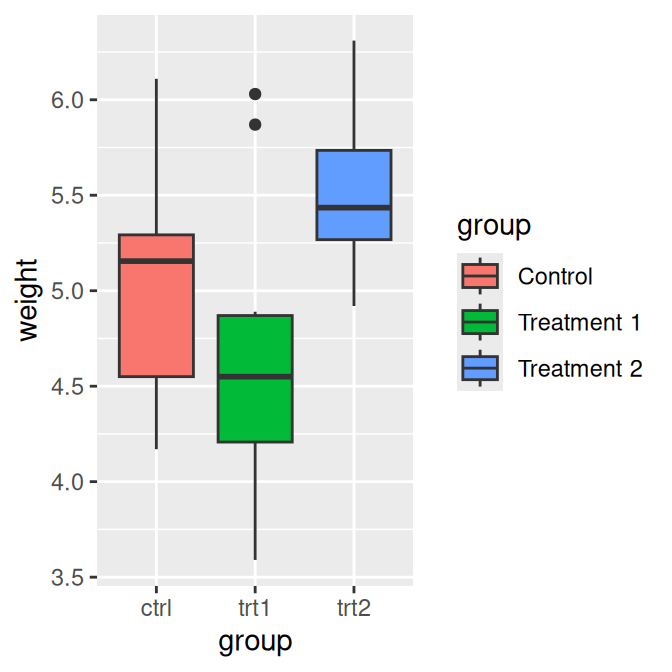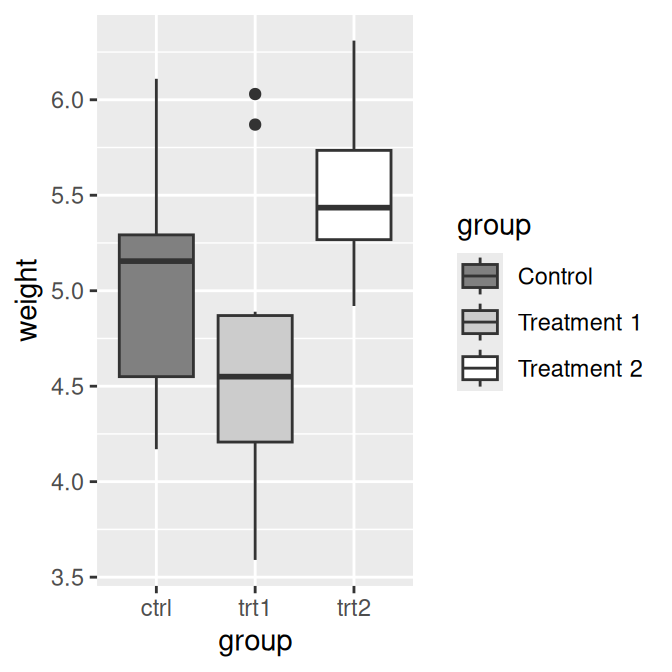Figure 10.13: Manually specified legend labels with the default discrete scale (left); Manually specified labels with a different scale (right)

If you are also changing the order of items in the legend, the labels are matched to the items by position. In this example we’ll change the item order, and make sure to set the labels in the same order (Figure 10.14):

``````pg_plot +
scale_fill_discrete(
limits = c("trt1", "trt2", "ctrl"),
labels = c("Treatment 1", "Treatment 2", "Control")
)``````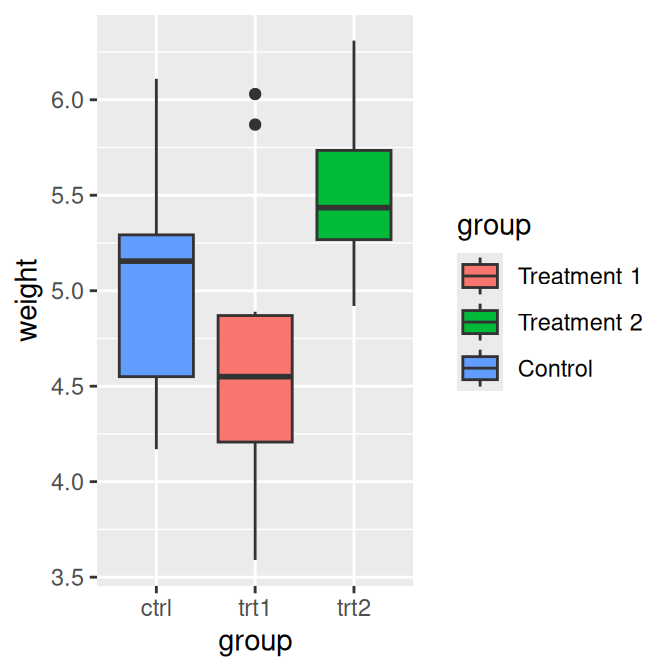Figure 10.14: Modified legend label order and manually specified labels (note that the x-axis labels and their order are unchanged)

If you have one variable mapped to two separate aesthetics, the default is to have a single legend that combines both. If you want to change the legend labels, you must change them for both scales; otherwise you will end up with two separate legends, as shown in Figure 10.15:

``````# Create the base plot
hw_plot <- ggplot(heightweight, aes(x = ageYear, y = heightIn, shape = sex, colour = sex)) +
geom_point()

hw_plot

# Change the labels for one scale
hw_plot +
scale_shape_discrete(labels = c("Female", "Male"))

# Change the labels for both scales
hw_plot +
scale_shape_discrete(labels = c("Female", "Male")) +
scale_colour_discrete(labels = c("Female", "Male"))``````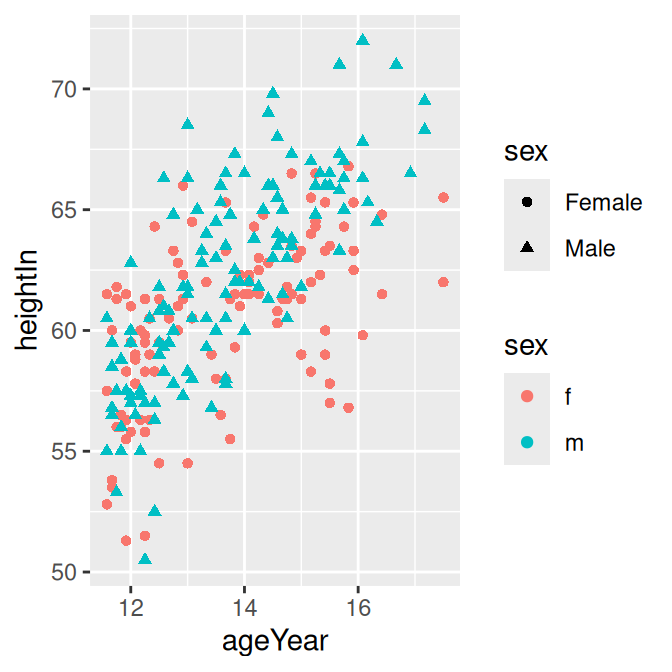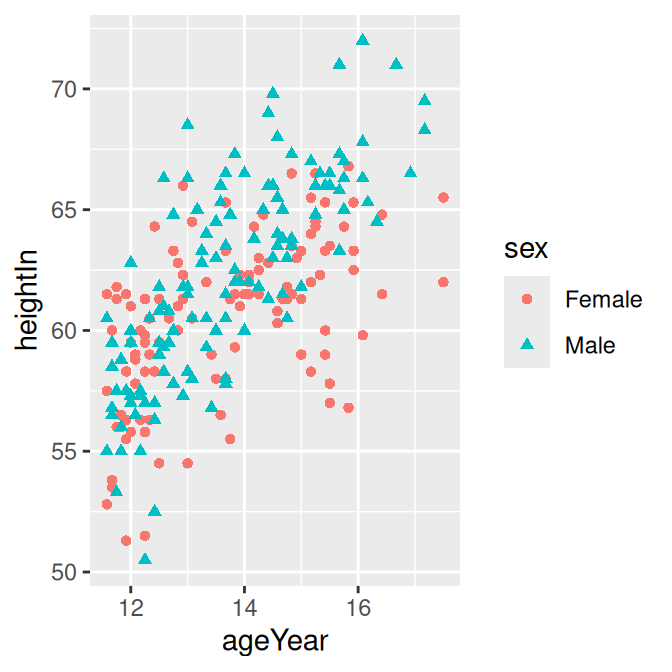Figure 10.15: A variable mapped to `shape` and `colour` (top left); With new labels for `shape` (top right); With new labels combining both `shape` and colour` (bottom)

Other commonly used scales with legends include:

• `scale_fill_discrete()`
• `scale_fill_hue()`
• `scale_fill_manual()`
• `scale_fill_grey()`
• `scale_fill_brewer()`
• `scale_colour_discrete()`
• `scale_colour_hue()`
• `scale_colour_manual()`
• `scale_colour_grey()`
• `scale_color_viridis_c()`
• `scale_color_viridis_d()`
• `scale_shape_manual()`
• `scale_linetype()`

By default, using `scale_fill_discrete()` is equivalent to using `scale_fill_hue()`; the same is true for color scales.

## 10.9 Changing the Appearance of Legend Labels

### 10.9.1 Problem

You want to change the appearance of labels in a legend.

### 10.9.2 Solution

Use `theme(legend.text=element_text())` (Figure 10.16):

``````# Create the base plot
pg_plot <- ggplot(PlantGrowth, aes(x = group, y = weight, fill = group)) +
geom_boxplot()

# Change the legend label appearance
pg_plot +
theme(legend.text = element_text(
face = "italic",
family = "Times",
colour = "red",
size = 14)
)``````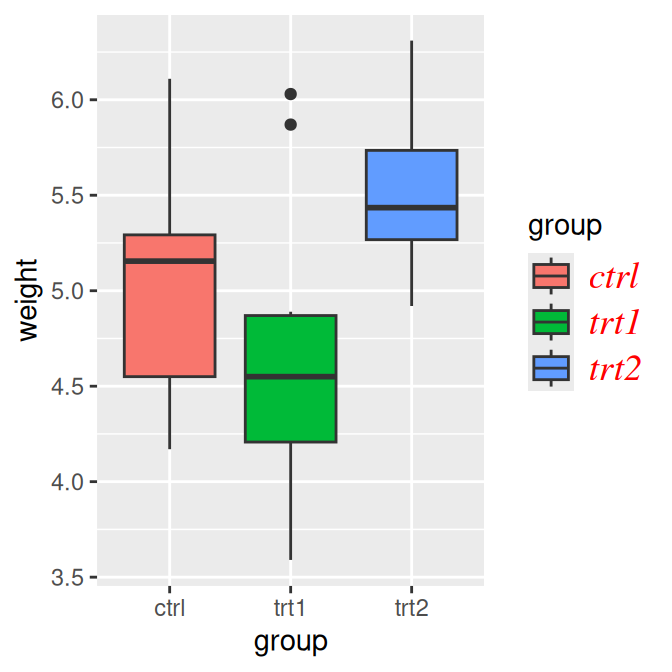Figure 10.16: Customized legend label appearance

### 10.9.3 Discussion

It’s also possible to specify the legend label appearance via `guides()`, although this method is a bit unwieldy. This has the same effect as the previous code:

``````# Changes the legend title text for the fill legend
pg_plot +
guides(fill = guide_legend(title.theme = element_text(
face = "italic",
family = "times",
colour = "red",
size = 14))
)``````

### 10.9.4 See Also

See Recipe 9.2 for more on controlling the appearance of text.

## 10.10 Using Labels with Multiple Lines of Text

### 10.10.1 Problem

You want to use legend labels that have more than one line of text.

### 10.10.2 Solution

Set the labels in the scale, using `\n` to represent a newline. In this example, we’ll use `scale_fill_discrete()` to control the legend for the fill scale (Figure 10.17, left):

``````pg_plot <- ggplot(PlantGrowth, aes(x = group, y = weight, fill = group)) +
geom_boxplot()

# Labels that have more than one line
pg_plot +
scale_fill_discrete(labels = c("Control", "Type 1\ntreatment", "Type 2\ntreatment"))``````

### 10.10.3 Discussion

As you can see in the version on the left in Figure 10.17, with the default settings the lines of text will run into each other when you use labels that have more than one line. To deal with this problem, you can increase the height of the legend keys and decrease the spacing between lines, using `theme()` (Figure 10.17, right). To do this, you will need to specify the height using the `unit()` function from the grid package:

``````library(grid)

pg_plot +
scale_fill_discrete(labels = c("Control", "Type 1\ntreatment", "Type 2\ntreatment")) +
theme(legend.text = element_text(lineheight = .8), legend.key.height = unit(1, "cm"))``````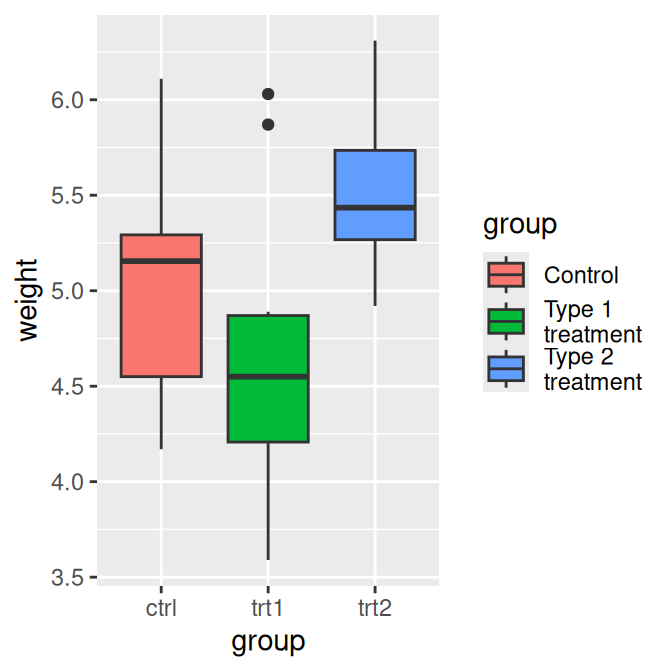Figure 10.17: Multiline legend labels (left); With increased key height and reduced line spacing (right)